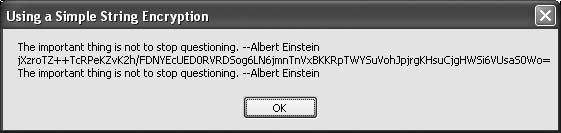# Recipe 5.33. Using a Simple String Encryption

#### Problem

You want to encrypt a string using a key. The encrypted result should be a displayable and printable string of standard ASCII characters.

#### Solution

Sample code folder: Chapter 05\EncryptString

The following short class defines a SimpleCrypt object containing shared functions for encrypting and decrypting a string. In addition to the string to be encrypted or decrypted, an integer is passed to each function to serve as the key:

` Public Class SimpleCrypt    Public Shared Function Encrypt(ByVal source As String, _          ByVal theKey As Integer) As String       ' ----- Encrypt a string.       Dim counter As Integer       Dim jumbleMethod As New Random(theKey)       Dim keySet(source.Length - 1) As Byte       Dim sourceBytes() As Byte = _          System.Text.Encoding.UTF8.GetBytes(source)       jumbleMethod.NextBytes(keySet)       For counter = 0 To sourceBytes.Length - 1          sourceBytes(counter) = _             sourceBytes(counter) Xor keySet(counter)       Next counter       Return Convert.ToBase64String(sourceBytes)    End Function    Public Shared Function Decrypt(ByVal source As String, _          ByVal theKey As Integer) As String       ' ----- Decrypt a previously encrypted string.       Dim counter As Integer       Dim jumbleMethod As New Random(theKey)       Dim sourceBytes() As Byte = _          Convert.FromBase64String(source)       Dim keySet(sourceBytes.Length - 1) As Byte       jumbleMethod.NextBytes(keySet)       For counter = 0 To sourceBytes.Length - 1          sourceBytes(counter) = _             sourceBytes(counter) Xor keySet(counter)       Next counter       Return System.Text.Encoding.UTF8.GetString(sourceBytes)    End Function End Class `

#### Discussion

The following code calls the shared functions of the SimpleCrypt class to encrypt a sample string using a key integer value of 123456789, and then decrypts the results using the same key:

` Dim quote As String = "The important thing is not to " & _    "stop questioning. --Albert Einstein" Dim myKey As Integer = 123456789 Dim encrypted As String = SimpleCrypt.Encrypt(quote, myKey) Dim decrypted As String = _    SimpleCrypt.Decrypt(encrypted, myKey) MsgBox(quote & vbNewLine & encrypted & vbNewLine & decrypted) `

The encryption function first converts the string to a byte array using UTF8 encoding. Each byte is then Xor'd with a predictable sequence of pseudorandom bytes seeded using the given key integer, and the resulting byte array is converted back to a string. Since this encrypted string likely contains ASCII characters in the range of control and nonprintable characters, the string is then converted to a slightly longer Base64 string comprised of displayable characters.

The decryption function reverses the order of these same steps. First, the Base64 string is converted to a byte array, and the same set of pseudorandom bytes is Xor'd with these bytes to recover the bytes of the original string. Figure 5-37 shows the original string, the encrypted version of this string using a key value of 123456789, and the string that results by decrypting this Base64 string using the same key. As expected, the original string is restored.

##### Figure 5-37. Encrypting and decrypting a string using a key integerThe Random object can return an array of pseudorandom bytes with any desired length. This lets the code generate the required number of bytes used in the Xor process with only one call to the Random object.

The supplied key is any integer value from 0 to the maximum value for signed integers, which is 2,147,483,647. You can use a negative integer, but the Random class will automatically take its absolute value as the seed.

With over two billion unique seeds, the average user won't be able to break this simple encryption easily. For quick, simple, relatively secure encryption for typical users, this class can serve you well. However, in cryptographic circles this level of encryption is considered dangerously poor, so be sure to check out Chapter 16 if you need to use something more serious and well tested by the cryptographic community.

See Chapter 16 for more encryption topics.Visual Basic 2005 Cookbook: Solutions for VB 2005 Programmers (Cookbooks (OReilly))
ISBN: 0596101775
EAN: 2147483647
Year: 2006
Pages: 400

Similar book on Amazon